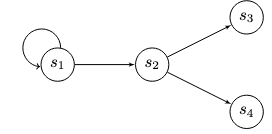# Transaction Tree#

A transaction tree is an extension of transaction chains where the code can contain if statements and simple loops, i.e., where some start and end nodes are the same. The following figure illustrates a transaction tree.An if statement is handled using the following pseudocode.

if (condition) { out.propositionBytes == state_3_code }
else { out.propositionBytes == state_4_code }


A simple loop is a special case of the if statement:

if (condition) { out.propositionBytes == state_2_code }
else { out.propositionBytes == SELF.propositionBytes }


Most useful contracts can be represented using branches and simple loops but no cycles (as shown by examples in the paper). Ergo can be used to create such contracts using UTXOs.

Next, we'll look at Transaction Graphs